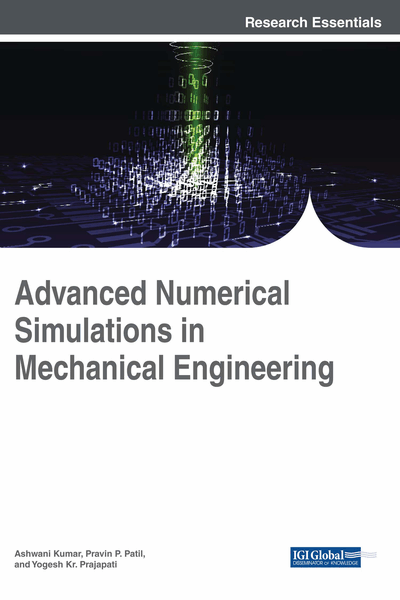# Mathematical Modeling of Five-Link Inverted Cart and Pendulum System

Ashwani Kharola (Graphic Era University, India)
DOI: 10.4018/978-1-5225-3722-9.ch008

## Abstract

This chapter describes a mathematical model and design structure of five-link inverted pendulum on cart. The system comprises of five rigid pendulums or links mounted on a mutable cart. The objective is to control all the five links at vertical upright position when cart is stationary at particular location. The study considered free-body-diagram (FBD) analysis of proposed system and applied Newton's second law of motion for deriving a mathematical model of proposed system. The derived governing equations of motion can be further used by researchers for developing a Matlab-Simulink model of five-link inverted pendulum system. The developed model can be further used for deriving equations of motions for n-link cart and pendulum system. Researchers can further apply various control techniques for control of proposed system.
Chapter Preview
Top